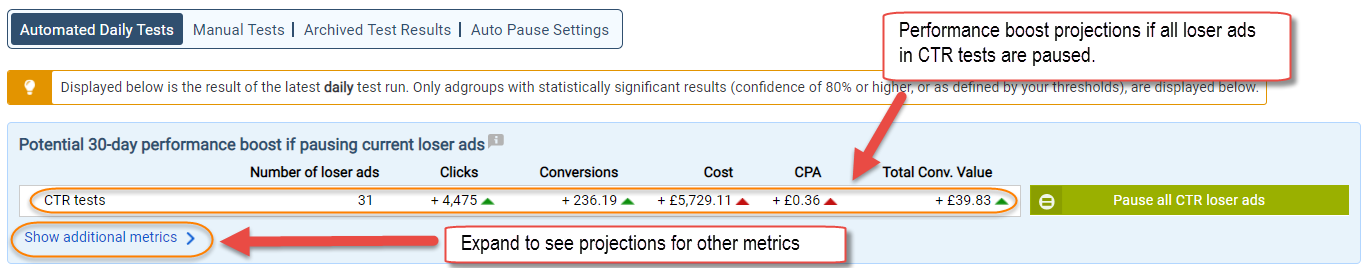1. What are the performance boost projections?

To help you understand the impact of pausing a loser Ad, Adalysis calculates (for every test result) the change that potentially can take place if the loser Ad were to be paused/deleted.

The performance change is calculated for 5 figures; clicks, conversions, cost, CPA and total conversion value.

The boost projection are shown in the following places:

1.1 For each test result1.2 Aggregated at the account or campaign level

Aggregated data shown for all currently available test results in the single-adgroup screen...Tip: The above example screenshot shows that if all 31 CTR loser ads are paused, you can potentially get 4,475 more clicks in the next 30 days.

2. How are they calculated?

To calculate the potential performance change projections, AdAlysis assumes that the total number of impressions all your Ads achieved in the last X days (where X is the date range used for the test) will remain the same over the next X days as well.

Assuming the loser Ad is paused, the projections are worked out as follows:

1. Assuming the loser Ad is no longer there, Adalysis splits the impressions of the loser Ad between the remaining Ads in the adgroup in the same proportion as their ad serving percentages relative to each other (see below example) .
2. The proportional increase in the impressions of each Ad is then used to calculate the proportional change in the clicks, conversions, cost, conversion value and CPA (assuming the CTR, CVR and CPC of each non-loser Ad stays the same).
3. The new totals are then compared to the old totals to find out what the change is.
4. Finally, the data is normalized to a 30 days period for consistency purposes.

The performance change projections are shown at each test result, as well as aggregated at the campaign and account level.

The same above principles apply to how the performance change is calculated for multi-adgroup tests.

ExampleIn this adgroup, the total impressions are 145,117.  The current totals of the remaining metrics are shown in the table below.

If the loser Ad were to be paused, the impressions of the winner Ad would be 145,117 instead of 64,833. Assuming CTR, CVR and CPC of the winning Ad remain the same, the proportional increase in the winning Ad performance is worked out:Finally, the data is normalized to a 30 days date range. However, since the test date range was 30 days (see screenshot), the above figures are displayed as is.

If there are more than 2 Ads in the adgroup, the impressions of the loser Ad are split between the winning Ads in the same proportion as their current Ad serving percentage.  Hence, in the below example, 7110 impressions are split between the 2 Ads in the ratio of 6191:6696 before the proportional increases are calculated.